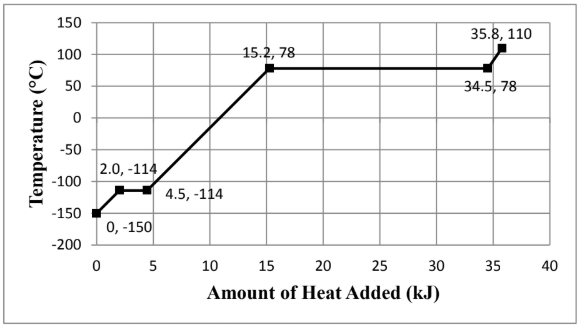# Problem: Consider the heating curve shown below for 22.86 grams of an unknown substance. You may assume constant pressure conditions. Note: the points are labeled as (amount of heat added, temperature). Calculate the enthalpy of fusion, ΔH fus, (in kJ/mol). Show all work and circle your final answer. The moles were identified to be 0.496 mol.

###### FREE Expert Solution

We are asked to the enthalpy of fusion, ΔH fus, (in kJ/mol) given the heating curve.

Phase changes occur on the horizontal parts of the curve since there are no temperature changes.

We go through the following steps to solve this problem:

Step 1. Calculate the heat to vaporize

Step 2. Calculate the molar heat of fusion###### Problem Details

Consider the heating curve shown below for 22.86 grams of an unknown substance. You may assume constant pressure conditions. Note: the points are labeled as (amount of heat added, temperature).Calculate the enthalpy of fusion, ΔH fus, (in kJ/mol). Show all work and circle your final answer. The moles were identified to be 0.496 mol.